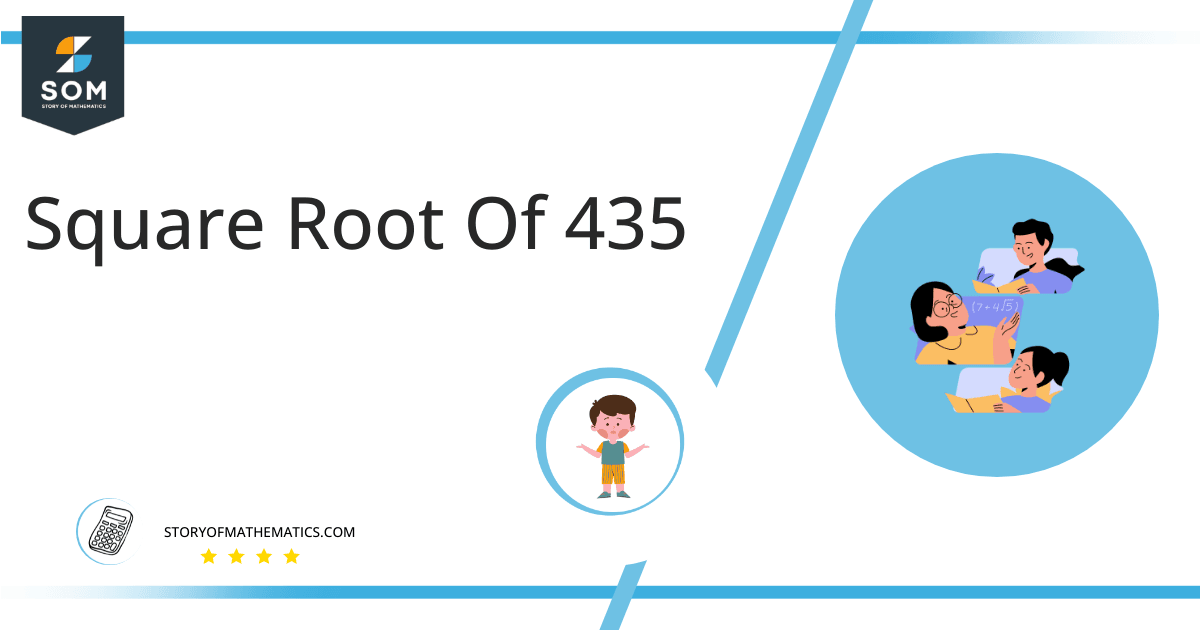# Square Root of 435 + Solution With Free Steps435 has a square root of 20.85. An integer that results from multiplying another integer by itself is known as a perfect square. Therefore, since 20.85 is an irrational number, we can conclude that 435 is not a perfect square. You will discover various methods for computing 435’s square root in this article.

In this article, we will analyze and find the square root of 435 using various mathematical techniques, such as the approximation method and the long division method.

## What Is the Square Root Of 435?

The square root of the number 435 is 20.85.

The square root can be defined as the quantity that can be doubled to produce the square of that similar quantity. In simple words, it can be explained as:

√435 = √(20.85 x 20.85)

√435 = √(20.85)$^2$

√435 = ±20.85

The square can be canceled with the square root as it is equivalent to 1/2; therefore, obtaining 20.85. Hence 20.85 is 435’s square root. The square root generates both positive and negative integers.

## How To Calculate the Square Root of 435?

You can calculate the square root of 435 using any of two vastly used techniques in mathematics; one is the Approximation technique, and the other is the Long Division method.

The symbol √ is interpreted as 435 raised to the power 1/2. So any number, when multiplied by itself, produces its square, and when the square root of any squared number is taken, it produces the actual number.

Let us discuss each of them to understand the concepts better.

### Square Root of 435 by Long Division Method

The process of long division is one of the most common methods used to find the square roots of a given number. It is easy to comprehend and provides more reliable and accurate answers. The long division method reduces a multi-digit number to its equal parts.

Learning how to find the square root of a number is easy with the long division method. All you need are five primary operations- divide, multiply, subtract, bring down or raise, then repeat.

Following are the simple steps that must be followed to find the square root of 435 using the long division method:

### Step 1

First, write the given number 435 in the division symbol, as shown in figure 1.

### Step 2

Starting from the right side of the number, divide the number 435 into pairs such as 35 and 4.

### Step 3

Now divide the digit 4 by a number, giving a number either 2 or less than 2. Therefore, in this case, the remainder is zero whereas the quotient is 2.

### Step 4

After this, bring down the next pair 35. Now the dividend is 035. To find the next divisor, we need to double our quotient obtained before. Doubling 2 gives 4; hence consider it as the next divisor.

### Step 5

Now pair 2 with another number to make a new divisor that results in $\leq$ 035 when multiplied with the divisor. If the number is not a perfect square, add pair of zeros to the right of the number before starting division.

### Step 6

Adding 0 to the divisor and multiplying 40 with 0 results in 000 < 235. The remainder obtained is m1. Move the next pair of zeros down and repeat the same process mentioned above.

### Step 7

Keep on repeating the same steps till the zero remainder is obtained or if the division process continues infinitely, solve to two decimal places.

### Step 8

The resulting quotient 20.85 is the square root of 435. Figure 1 given below shows the long division process in detail:Figure 1

### Square Root by Approximation Method

The approximation method involves guessing the square root of the non-perfect square number by dividing it by the perfect square lesser or greater than that number and taking the average.

The given detailed steps must be followed to find the square root of 435 using the approximation technique.

### Step 1

Consider a perfect square number of 20 less than 435.

### Step 2

Now divide 435 by 20.

435 ÷ 20 = 21.75

### Step 3

Now take the average of 20 and 21.75. The resulting number is approximately equivalent to the square root of 435.

(435 + 21.75) ÷ 2 =20.85

### Important points

• The number 435 is not a perfect square.
• The number 435 is an irrational number.
• The number 435 can be split into its prime factorization.

## Is Square Root of 435 a Perfect Square?

The number 435 is  not a perfect square. A number is a perfect square if it splits into two equal parts or identical whole numbers. If a number is a perfect square, it is also rational.

A number expressed in p/q form is called a rational number. All the natural numbers are rational. A square root of a perfect square is a whole number; therefore, a perfect square is a rational number.

A number that is not a perfect square is irrational as it is a decimal number. As far as 435 is concerned, it not a perfect square. It can be proved as below:

Factorization of 435 results in 5 x 87.

Taking the square root of the above expression gives:

= √(5 x 87)

= (5 x 87)$^{1/2}$

= 20.85

This shows that 435 is not a perfect square as it has decimal places; hence it is an irrational number.

Therefore the above discussion proves that the square root of 435 is equivalent to 20.85.Images/mathematical drawings are created with GeoGebra.